# 18. The grain of a wooden member forms an angle of 15° with the vertical. For the state of stress shown, determine (a) the inplane shearing stress parallel to the grain, (b) the normal stress perpendicular to the grain.

Question-AnswerCategory: Strength of Materials18. The grain of a wooden member forms an angle of 15° with the vertical. For the state of stress shown, determine (a) the inplane shearing stress parallel to the grain, (b) the normal stress perpendicular to the grain.

The grain of a wooden member forms an angle of 15° with the vertical. For the state of stress shown, determine (a) the inplane shearing stress parallel to the grain, (b) the normal stress perpendicular to the grain.Step No: 1

a)
Draw the diagram representing the stresses acting on the planes.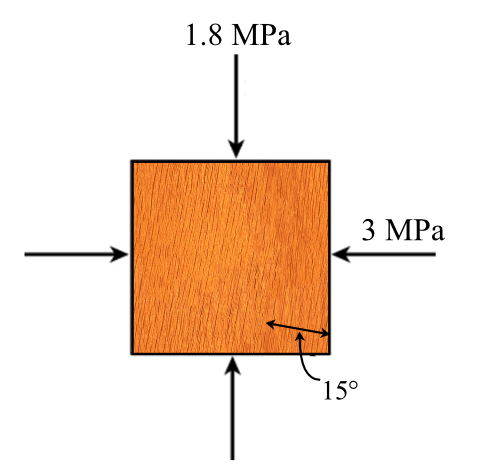Step No: 2

As there is no shear stress acting on the xy plane, the shear stress is equal to zero.Calculate the in-plane shearing stress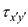parallel to the grain by using the equation.Here,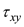is the shear stress acting along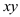plane,is the direct stress acting along x direction, andis the direct stress acting along y direction.
Substitutefor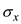forfor, and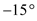for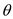.Therefore, the in-plane shearing stress parallel to the grain is.

Step No: 3

b)
Calculate the normal stressperpendicular to the grain by using the formula.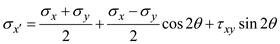Substituteforfor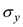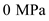for, andfor.Therefore, the normal stress acting perpendicular to the grain is.